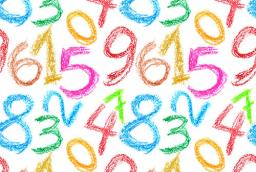# Difference 3300

The difference between five and three times the number, what I think, is three greater than this number. What number do I think?

x =  3

### Step-by-step explanation:

5x-3x = x+3

5·x-3·x = x+3

x = 3

x = 3/1 = 3

x = 3

Our simple equation calculator calculates it.Did you find an error or inaccuracy? Feel free to write us. Thank you!

Tips for related online calculators
Do you have a linear equation or system of equations and looking for its solution? Or do you have a quadratic equation?